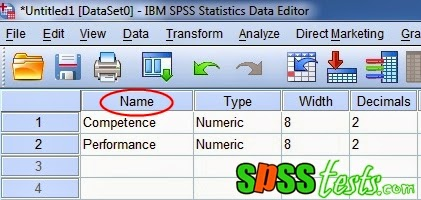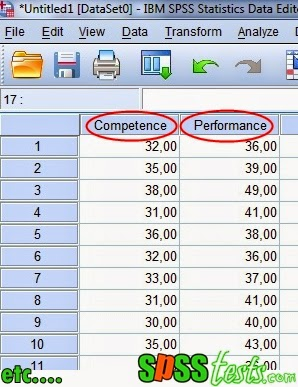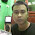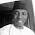# Step By Step to Test Linearity Using SPSS

Step By Step to Test Linearity Using SPSS | Linearity test aims to determine the relationship between independent variables and the dependent variable is linear or not. The linearity test is a requirement in the correlation and linear regression analysis. Good research in the regression model there should be a linear relationship between the free variable and dependent variable.

Decision-making process in the Linearity Test
1. If the value sig. Deviation from Linearity> 0.05, then the relationship between the independent variables are linearly dependent.
2. If the value sig. Deviation from Linearity <0.05, then the relationship between independent variables with the dependent is not linear.

Example Case in Linearity Test

A company manager wants to know whether there is a linear relationship between competence with Employee Performance. For the manager of the collected data Competence and Performance of 40 samples of employees. The research data as shown below.Step By Step to Test Linearity Using SPSS

1. Turn on the SPSS program and select the Variable View, next, in the Name write Competency and Performance. Ignore the other options.2. The next step, click the Data View and enter research data in accordance with the competence and performance variables.3. Next, from the SPSS menu select Analyze, and then click Compare Means and then click Means…4. Then a new dialog box will appear with the name of Means, and then enter into the box Independent list Competency and Performance Dependent list box, and then click on Options5. The dialog box appears with the name of Means:Options, on the Statistics for First Layer, select Test for Linearity, and then click Continue6. The last step clicks on Ok to terminate the command, after which it will appear SPSS output, as follows:Interpretation of Test Results Output Linearity

Based on the ANOVA Output Table, value sig. Deviation from Linearity of 0.423> 0.05, it can be concluded that there is a linear relationship between the variables of Competence with Employee Performance.

Next: Kolmogorov-Smirnov Normality Test in Regression Models

[Keywords: How to test Linearity Test Using SPSS | How To Perform Linearity Test in SPSS | Step By Step To Test Linearity Using SPSS | Tutorial Linearity Test in SPSS with SPSS Software Complete]
[Image: Data SPSS Version 21]
[Source: Summarized from various sources]

### 4 Responses to "Step By Step to Test Linearity Using SPSS"

1.thank you for your invaluable service

1.Thank you again

2.This is really helpful.

1.